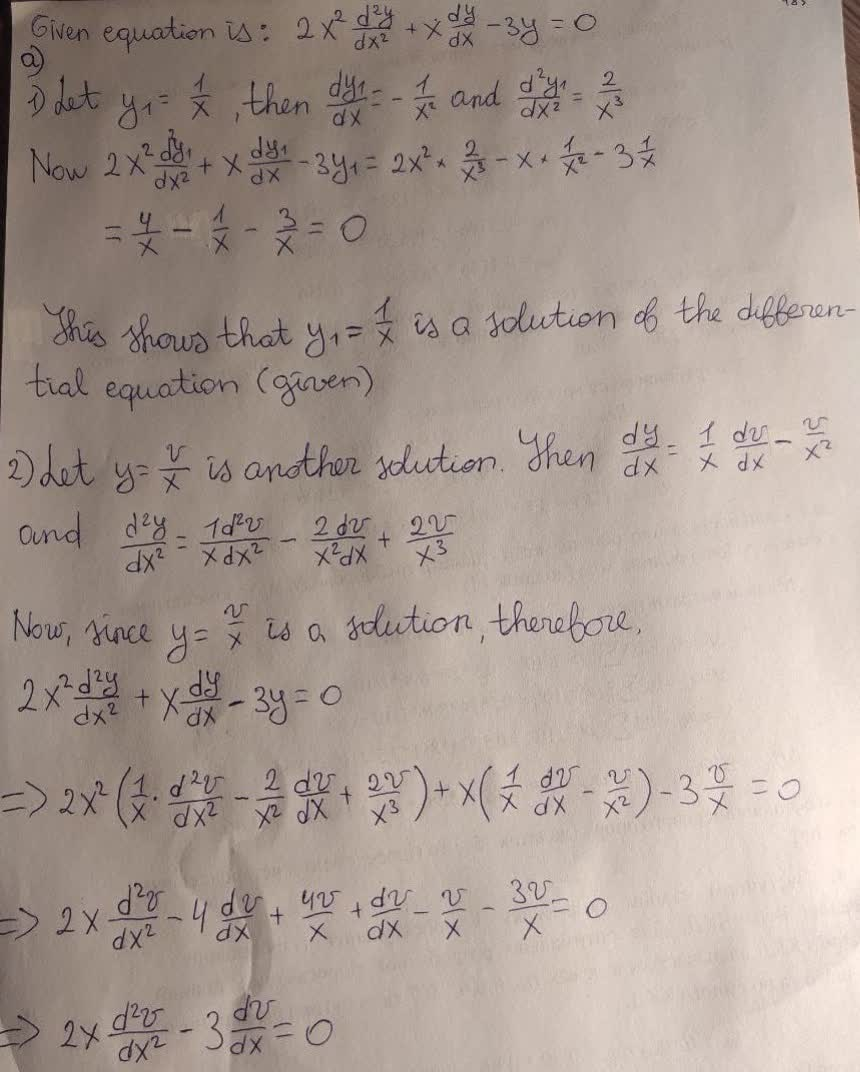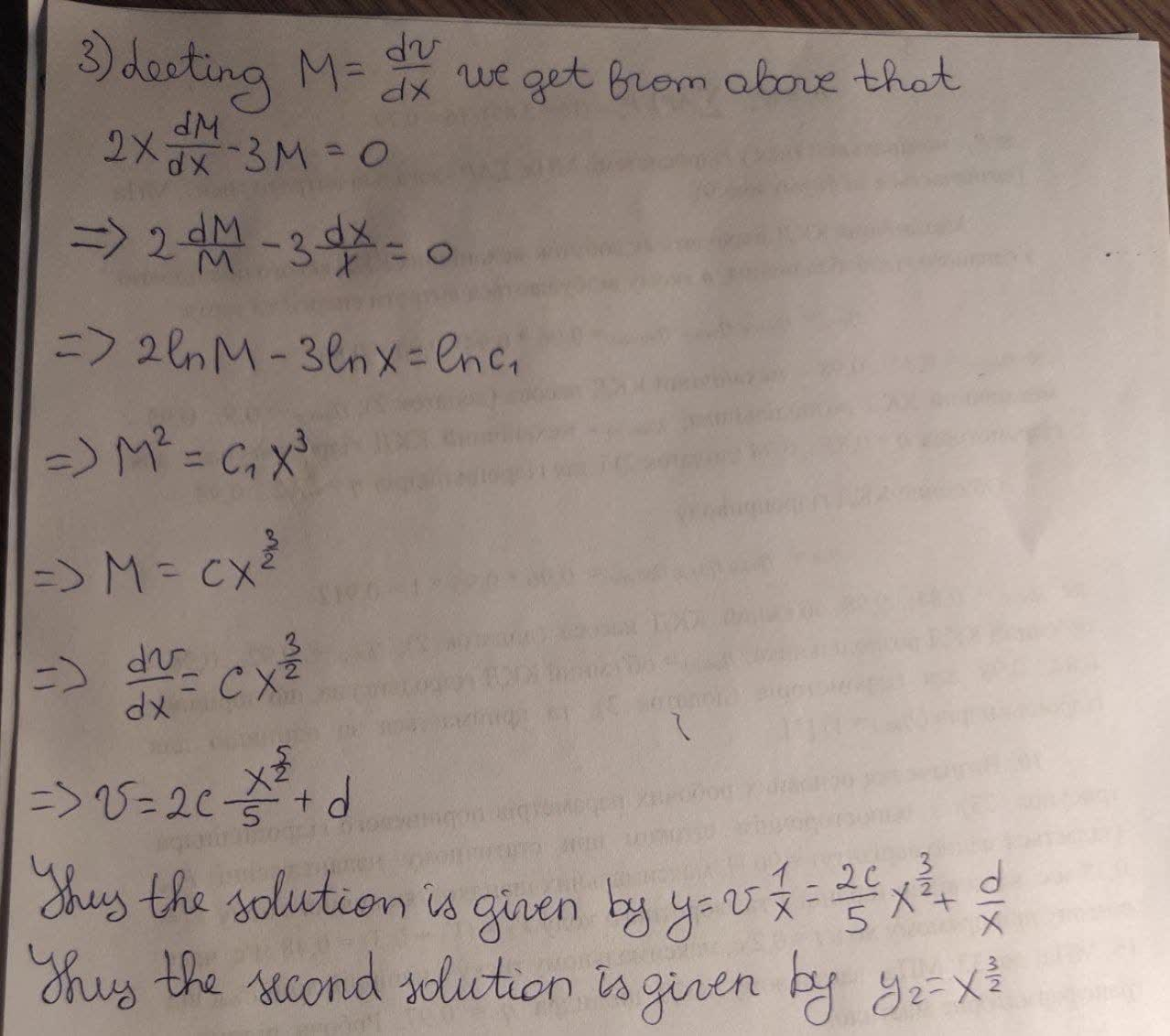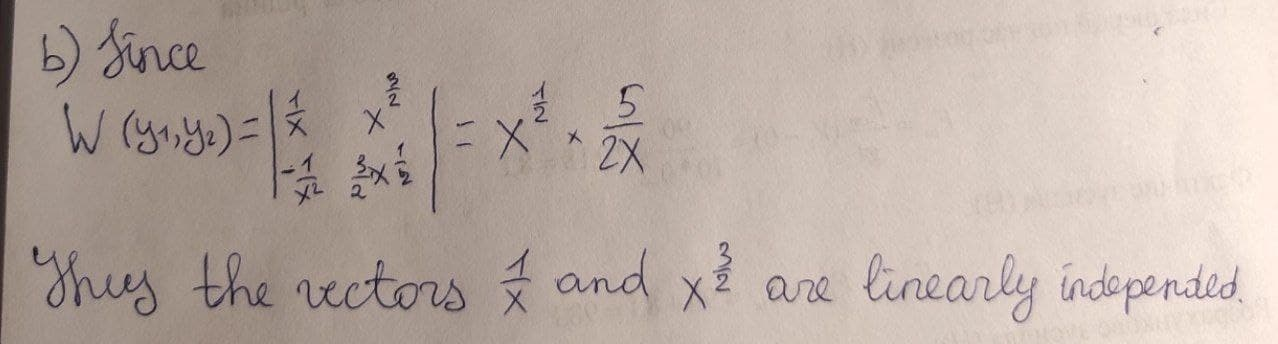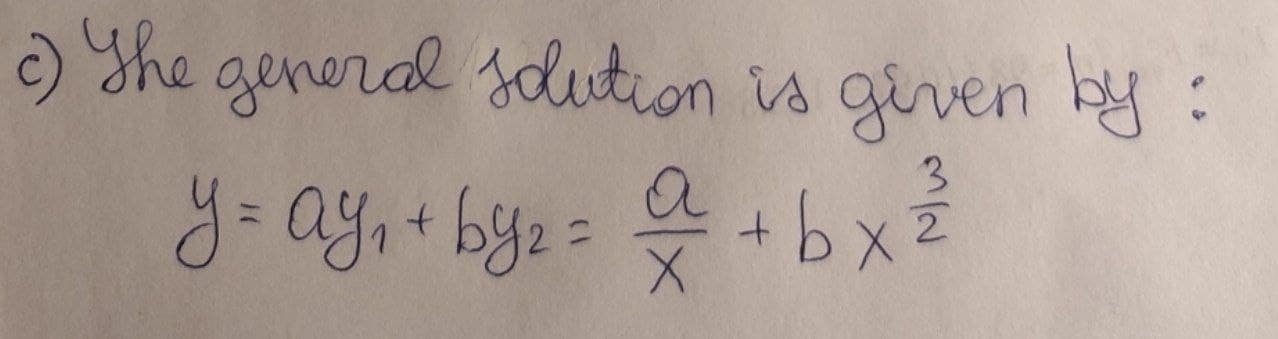# given y=1/x is a solution 2x^2 d2y/dx + xdy/dx - 3y = 0 , x>0 a) Find a linearly independent solution by reduction the order approach b) Show that 2 solutions are linearly independent c) Write a general solutioniohanetc 2021-02-26 Answered
given $y=\frac{1}{x}$ is a solution $2{x}^{2}d2\frac{y}{dx}+x\frac{dy}{dx}-3y=0,x>0$
a) Find a linearly independent solution by reduction the order approach
b) Show that 2 solutions are linearly independent
c) Write a general solution
You can still ask an expert for help

• Questions are typically answered in as fast as 30 minutes

Solve your problem for the price of one coffee

• Math expert for every subject
• Pay only if we can solve it2k1enyvp
I think this is the correct answer: